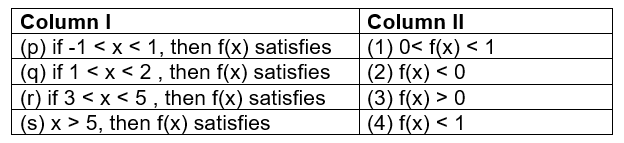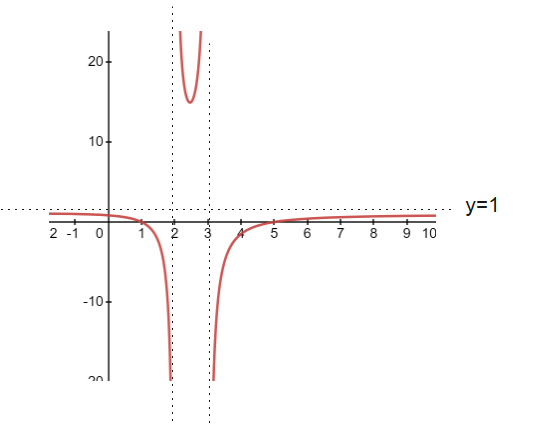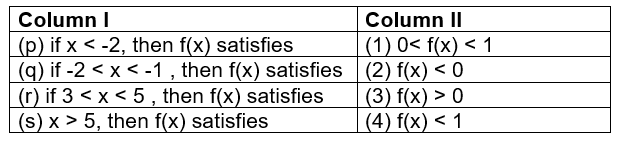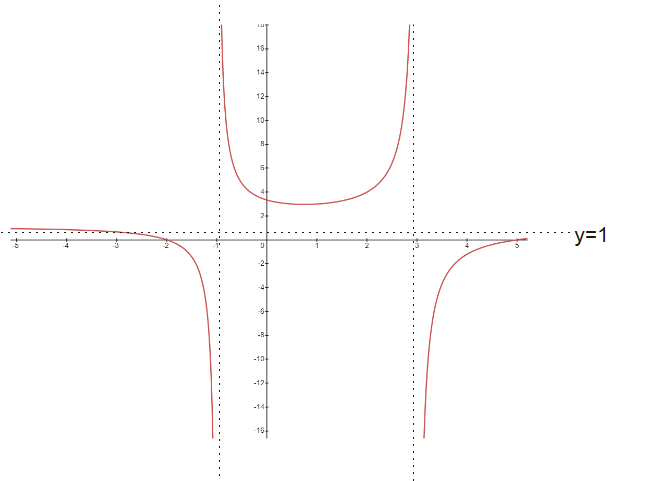# JEE Main and Advanced questions on relations and functions

In this page we have JEE Main and Advanced questions on relations and functions. Hope you like them and do not forget to like , social share and comment at the end of the page.

## Multiple Choice Questions

Question 1
If the function f: R-> R defined as $f(x) =|x|(x -sin x)$, then which of the following statement is true
(a) f is bijective
(b) f is one-one but not Onto
(c) f is onto but not one-one
(d) f is neither one-one nor onto

Question 2
If the function f:$[1,\infty)$ -> $[2,\infy)$ defined as $f(x) =x + \frac {1}{x}$, then $f^{-1}$ x is
(a) $\frac {x}{1+x^2}$
(b) $x + \frac {1}{x}$
(c) $\frac {x + \sqrt {x^2 -4}}{2}$
(d) $\frac {x - \sqrt {x^2 -4}}{2}$

(c)
$y=x + \frac {1}{x}$ $xy=x^2 +1$ $x^2 -xy + 1=0$ $x = \frac {y \pm \sqrt {y^2 -4}}{2}$ Here checking the domain and range $x= \frac {y + \sqrt {y^2 -4}}{2}$

Question 3
The function $f:[0,\infty)$ -> R given by $f(x)= \frac {x}{x+1}$ is
(a) one -one and onto
(b) one-one but not onto
(c) onto but not one-one
(d) Neither one-one nor onto

$f(x)= \frac {x}{x+1}$
$\frac {df(x)}{dx}= \frac {1}{(x+1)^2}$
This is positive, So f(x) is a increasing function and it is one-one function
for onto
$y=\frac {x}{x+1}$
$x = \frac {y}{1-y}$
So y cannot be 1,So Range is subset of codomain
Hence not onto function

Question 4
if $g(f(x))=sin^2 x$ and $f(g(x)) =(sin \sqrt x)^2$ then
(a) $f(x) =x^2$ and $g(x) = sin \sqrt x$
(b) $f(x) =sinx$ and $g(x) = |x|$
(c) $f(x) =sin^2 x$ and $g(x) = \sqrt x$
(d) f and g cannot be determined

Self explanatory

Question 5
The function $f(x)=[x] sin (\frac {\pi}{[x+1]})$ where [.] denotes the greatest integer function. The domain of the function f is
(a) $(-\infty ,\infty) - {-1}$
(b) $[0 ,\infty)$
(c) $(-\infty ,-1)$
(d) $(-\infty ,-1) \cup [0, \infty)$

$[x+1] \neq 0$
Hence domain is $(-\infty ,-1) \cup [0, \infty)$

Question 6
The domain of the function $f(x)=\frac {log_2 (x+3)}{x^2 + 3x +2}$?
(a) $R - {-1,-2,-3}$
(b) $R - {-1,-2}$
(c) $(-2,\infty)$
(d) $(-3, \infty) - {-1,-2}$

Here x+ 3 > 0 or x > -3
also $x^2 + 3x +2= (x+1)(x+2)$,so x cannot take the value -1 and -2
Hence the domain is $(-3, \infty) - {-1,-2}$

Question 7
Consider the below two relations A= {(x,y)|x,y are real numbers and x=wy for some rational number}
B= {(m/n,p/q)|m,n, p and q are integers such that n and q are not zero and qm=pn (a) A is a equivalence relation but B is not
(b) A and B both are equivalence relation
(c) A is not a equivalence relation but B is a equivalence relation

(d) A and B both are not equivalence relations

for A
xAy => x=wy
yAx => y=wx
and they are not equal, so it is not symmetric
For B
$\frac {m}{n} A \frac {m}{n}$ = mn=mn which is true for all m,n in integers
Hence Reflexive
if $\frac {m}{n} A \frac {p}{q}$ => mq=pn
This can be written s
np=mq i.e $\frac {p}{q} A \frac {m}{n}$
Hence symmetric
Now if $\frac {m}{n} A \frac {p}{q}$ => mq=pn and $\frac {p}{q} A \frac {r}{s}$ => ps=rq or rq=ps
then
(qm)(ps)= (pn)(rq)
ms=nr
ie.$\frac {m}{n} A \frac {r}{s}$
Hence transitive
So B is a equivalence relations
hence (c) is the correct answer

Question 8
let $f(x) = \frac {1}{\sqrt {|x| - x}}$, the domain of f(x) is
(a) $(-\infty ,\infty)$
(b) $(-\infty ,0)$
(c) $(-\infty ,\infty) - {0}$
(d) $(0 ,\infty) Answer Answer is (b) as |x| - x > 0 or |x| >x so x< 0 Question 9 Let f: R -> [-1/2,1/2] and$f(x) =\frac {x}{x^2 +1}$then f(x) is (a) one-one but not onto (b) onto but not one -one (c) one-one and onto (d) neither one-one nor onto Answer (b) Question 10 If$a \in R$and the equation$-3(x-[x])^2+2(x−[x])+a^2=0$(where [x] denotes the greatest integer ) has a no integral solution , then all possible values of a lie in the interval (a)(1,2) (b)(-2,-1) (c)$(-1,0) \cup (0,1)$(d)$(-2,0) \cup (0,2)$Answer$-3(x-[x])^2+2(x−[x])+a^2=0$let t=x-[x]. Now 0 Now equation becomes$-3(t)^2+2(t)+a^2=03t^2 -2t-a^2=0t=\frac { 2 \pm \sqrt {4 + 12a^2}}{6} = \frac {1 \pm \sqrt {1 + 3a^2}}{3}$it can be positive only as otherwise it will be lessor than t<0$t= \frac {1 + \sqrt {1 + 3a^2}}{3}$Now$\frac {1 + \sqrt {1 + 3a^2}}{3} < 1\sqrt {1 + 3a^2} < 21 + 3a^2 < 4a^2 < 1$Also a cannot be zero as then it will have integral solutions So answer is (c) ## Fill in the blanks Question 11 (i) Let f :[0,1] -> R be defined by$f(x) = \frac {4^x}{4^x +2}$Then the value of$f(\frac {1}{40}) + f(\frac {2}{40}) + f(\frac {3}{40}) + .............. + f(\frac {39}{40})$is ______ (ii) if$f(x)= \frac {1}{2} (sin^2 x + sin^2(x + \pi/3) + cosx cos(x + \pi/3))$and g(5/8) =1, then g(f(x)) is _____ (iii) The domain of the function$cos^{-1} (log_e (\frac {x}{x-1}))$is _______ Answer (i) 39/2 Here$f(x) = \frac {4^x}{4^x +2}f(1-x) = \frac {2}{4^x +2}$So$ f(x) + f(1-x)=1$Now$f(\frac {1}{40}) + f(\frac {2}{40}) + f(\frac {3}{40}) + .............. + f(\frac {39}{40})= \frac {1}{2} ( \sum_{k=1}^{k=39} f(\frac {k}{40}) + \sum_{k=1}^{k=39} f(\frac {k}{40})= \frac {1}{2} ( \sum_{k=1}^{k=39} f(\frac {k}{40}) + \sum_{k=39}^{k=1} f(\frac {k}{40})= \frac {1}{2} [\sum_{k=1}^{k=39} f(\frac {k}{40}) + f(\frac {40 -k}{40})] =\frac {39}{2}$(ii) 1 f(x)= \frac {1}{2} (sin^2 x + sin^2(x + \pi/3) + cosx cos(x + \pi/3))$
Applying sin (A+B) and cos(A+B) identities, we get
f(x) =5/8
hence g(f(x))=1
(iii) $(-\infty , -\frac {1}{e-1}] \cup [\frac {e}{e-1} , \infty)$

## Match the column

Question 12
Let $f(x)=\frac {x^2 -6x +5}{x^2 -5x + 6}$
Then match the column A and BThe graph of the function is given below
$f(x)=\frac {x^2 -6x +5}{x^2 -5x + 6}=\frac {(x-1)(x-5)}{(x-2)(x-3)}$(p) if 0< x <1 then 0 (q) if 1 < x < 2, then f(x) < 0, then 2,4 is the answer
(r) if 3 < x < 5, then f(x) < 0, then 2,4 is the answer
(s) if x> 5 then 0

Question 13
Let $f(x)=\frac {x^2 -3x -10}{x^2 -2x -3}$
Then match the column A and BThe graph of the function is given below
$f(x)=\frac {x^2 -3x -10}{x^2 -2x -3}=\frac {(x+2)(x-5)}{(x+1)(x-3)}$(p) if x < -2 then 0 (q) if -2 < x < -1, then f(x) < 0, then 2,4 is the answer
(r) if 3 < x < 5, then f(x) < 0, then 2,4 is the answer
(s) if x> 5 then 0

## True and False

Question 14
(i) The function $f(x)=sin(log(x + \sqrt {x^2 +1}))$ is odd function
(ii)If the function $f:[1, \infty) -> [1, \infty)$ is defined by $f(x)=2^{x(x−1)}, then$f^{−1}(x)$is given as$\frac {1}{2}[1 + \sqrt {1+4log_2 x}]\$
(iii) The relation R on the set A = {1, 2, 3} defined as R = {{1, 1), (1, 2), (2, 1), (3, 3)} is reflexive, symmetric and transitive.

(i) True
(ii) True
(iii) False

Go back to Class 12 Main Page using below links